# Log24

## Thursday, April 16, 2015

### National Library Week

Filed under: General,Geometry — Tags: , — m759 @ 7:00 PM

"Celebrate National Library Week 2015 (April 12-18, 2015)
with the theme "Unlimited possibilities @ your library®."

A page from Princeton University Press on March 18, 2012:

… "mathematics and narrative…." (top of page xvii).

I prefer the interplay of Euclidean  and Galois  mathematics.

## Tuesday, November 25, 2014

### Euclidean-Galois Interplay

Filed under: General,Geometry — Tags: , — m759 @ 11:00 AM

For previous remarks on this topic, as it relates to
symmetry axes of the cube, see previous posts tagged Interplay.

The above posts discuss, among other things, the Galois
projective plane of order 3, with 13 points and 13 lines.

These Galois points and lines may be modeled in Euclidean geometry
by the 13 symmetry axes and the 13 rotation planes
of the Euclidean cube. They may also be modeled in Galois geometry
by subsets of the 3x3x3 Galois cube (vector 3-space over GF(3)).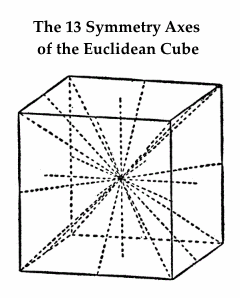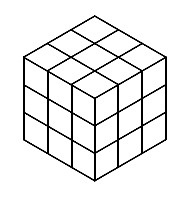The 3×3×3 Galois Cube

Exercise: Is there any such analogy between the 31 points of the
order-5 Galois projective plane and the 31 symmetry axes of the
Euclidean dodecahedron and icosahedron? Also, how may the
31 projective points  be naturally pictured as lines  within the
5x5x5 Galois cube (vector 3-space over GF(5))?

Update of Nov. 30, 2014 —

For background to the above exercise, see
pp. 16-17 of A Geometrical Picture Book ,
by Burkard Polster (Springer, 1998), esp.
the citation to a 1983 article by Lemay.

## Sunday, June 8, 2014

### Vide

Filed under: General,Geometry — Tags: , , — m759 @ 10:00 AM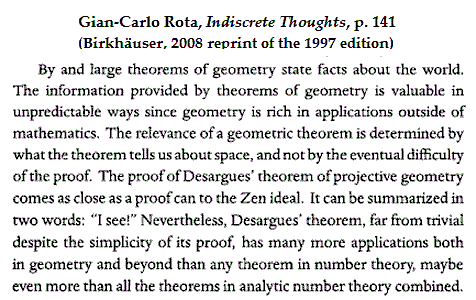“The relevance of a geometric theorem is determined by what the theorem
tells us about space, and not by the eventual difficulty of the proof.”

— Gian-Carlo Rota discussing the theorem of Desargues

What space  tells us about the theorem :

In the simplest case of a projective space  (as opposed to a plane ),
there are 15 points and 35 lines: 15 Göpel  lines and 20 Rosenhain  lines.*
The theorem of Desargues in this simplest case is essentially a symmetry
within the set of 20 Rosenhain lines. The symmetry, a reflection
about the main diagonal in the square model of this space, interchanges
10 horizontally oriented (row-based) lines with 10 corresponding
vertically oriented (column-based) lines.

* Update of June 9: For a more traditional nomenclature, see (for instance)
R. Shaw, 1995.  The “simplest case” link above was added to point out that
the two types of lines named are derived from a natural symplectic polarity
in the space. The square model of the space, apparently first described in
notes written in October and December, 1978, makes this polarity clearly visible: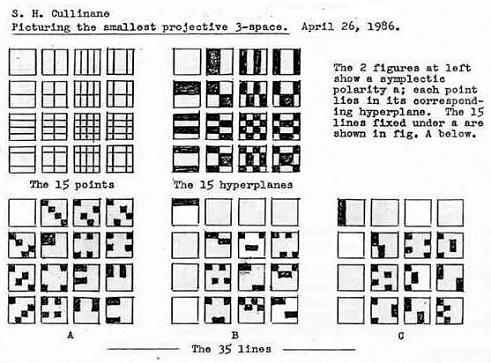## Tuesday, August 6, 2013

### Desargues via Galois

Filed under: General,Geometry — Tags: , — m759 @ 5:12 PM

The following image gives a brief description
of the geometry discussed in last spring's
Classical Geometry in Light of Galois Geometry.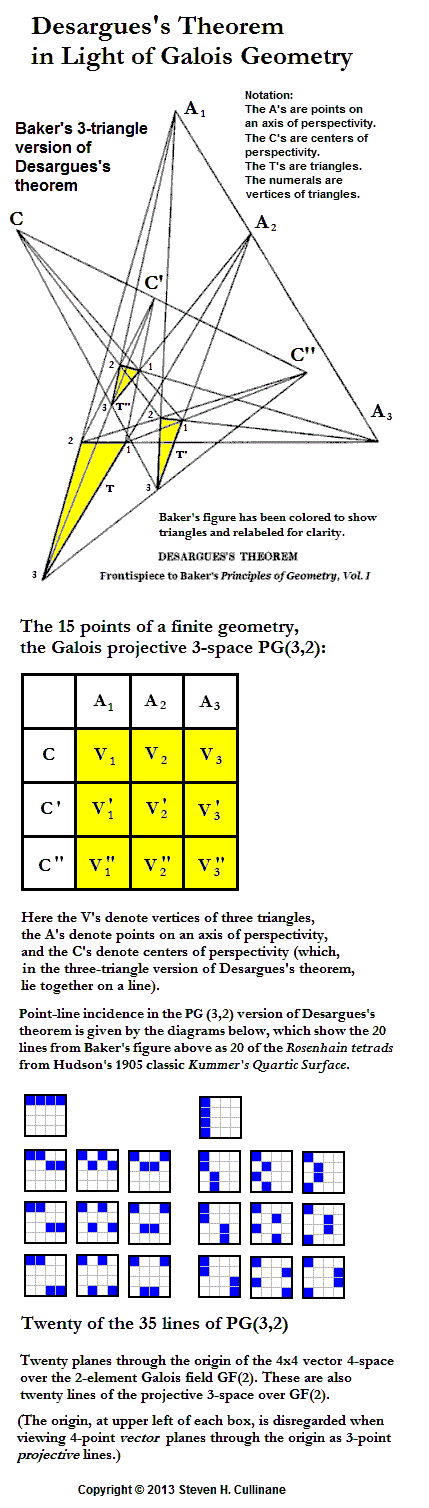## Monday, June 21, 2010

### Cube Spaces

Filed under: General,Geometry — Tags: — m759 @ 11:30 AM

Cubic models of finite geometries
display an interplay between
Euclidean and Galois geometry.

Example 1— The 2×2×2 Cube—

also known as the eightfold  cube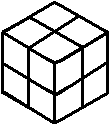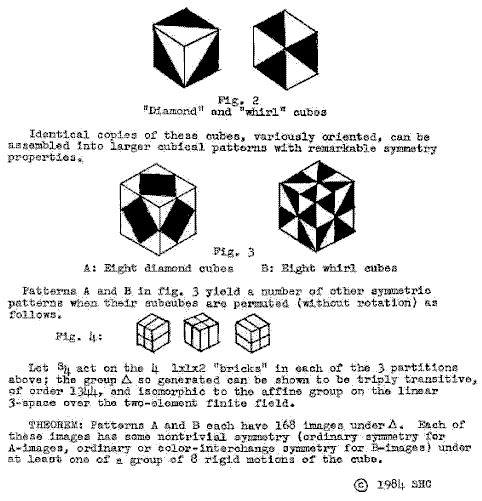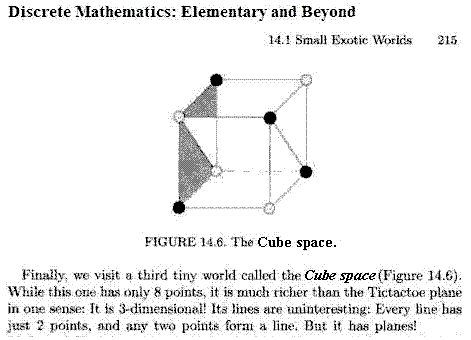Lovasz et al. go on to describe the same group actions
as in the 1984 note, without attribution.

Example 2— The 3×3×3 Cube

A note from 1985 describing group actions on a 3×3 plane array—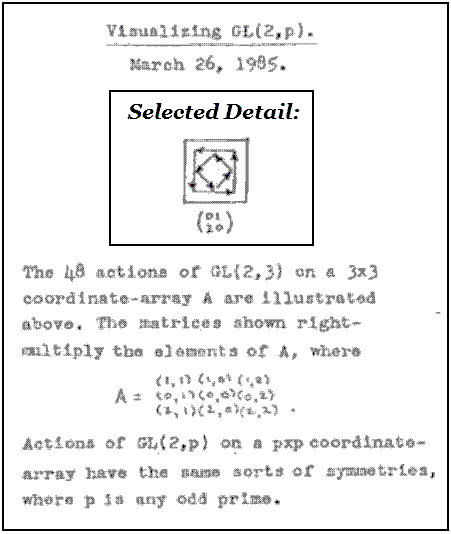Undated software by Ed Pegg Jr. displays
group actions on a 3×3×3 cube that extend the
3×3 group actions from 1985 described above—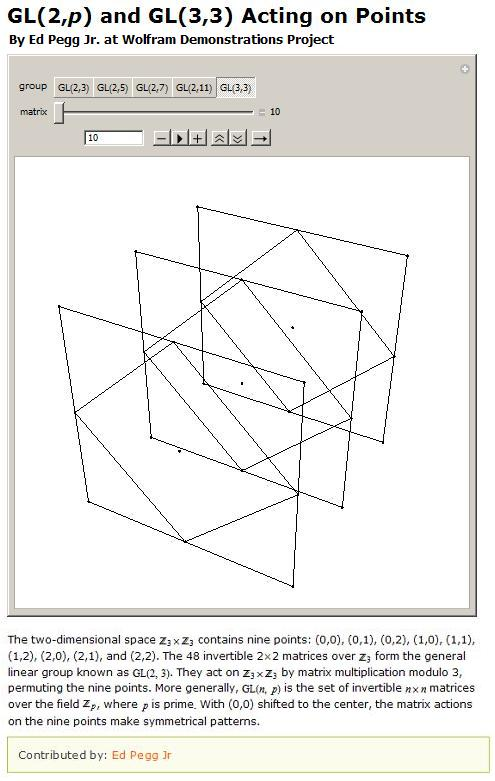Pegg gives no reference to the 1985 work on group actions.

Example 3— The 4×4×4 Cube

A note from 27 years ago today—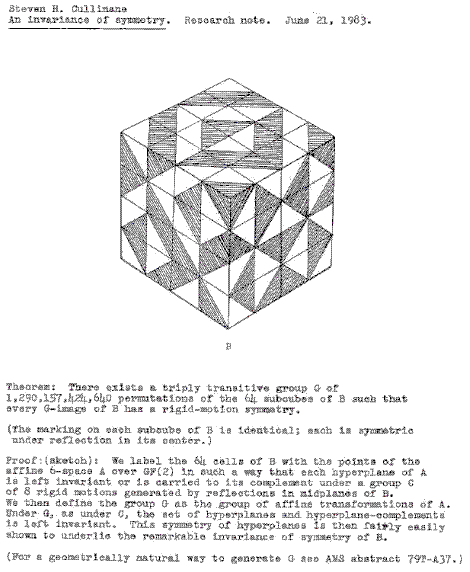As far as I know, this version of the
group-actions theorem has not yet been ripped off.

## Saturday, February 27, 2010

### Cubist Geometries

Filed under: General,Geometry — Tags: , — m759 @ 2:01 PM

"The cube has…13 axes of symmetry:
6 C2 (axes joining midpoints of opposite edges),
4 C3 (space diagonals), and
3C4 (axes joining opposite face centroids)."
–Wolfram MathWorld article on the cube

These 13 symmetry axes can be used to illustrate the interplay between Euclidean and Galois geometry in a cubic model of the 13-point Galois plane.

The geometer's 3×3×3 cube–
27 separate subcubes unconnected
by any Rubik-like mechanism–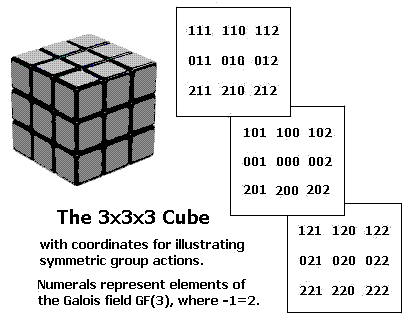The 13 symmetry axes of the (Euclidean) cube–
exactly one axis for each pair of opposite
subcubes in the (Galois) 3×3×3 cube–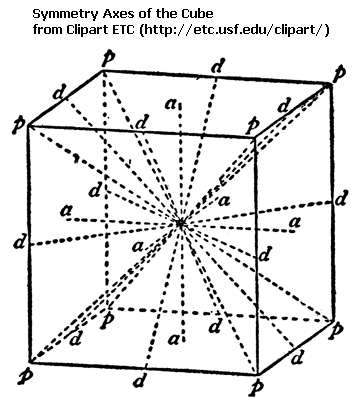A closely related structure–
the finite projective plane
with 13 points and 13 lines–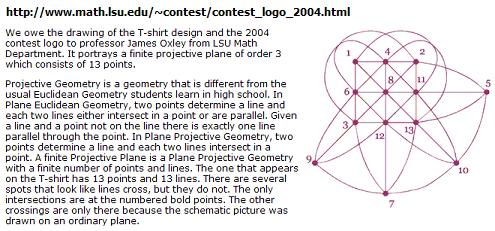A later version of the 13-point plane
by Ed Pegg Jr.–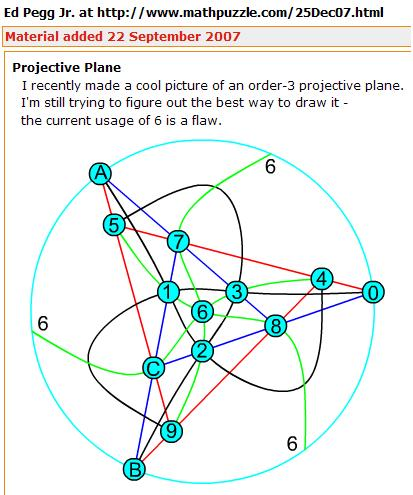A group action on the 3×3×3 cube
as illustrated by a Wolfram program
by Ed Pegg Jr. (undated, but closely
related to a March 26, 1985 note
by Steven H. Cullinane)–The above images tell a story of sorts.
The moral of the story–

Galois projective geometries can be viewed
in the context of the larger affine geometries
from which they are derived.

The standard definition of points in a Galois projective plane is that they are lines through the (arbitrarily chosen) origin in a corresponding affine 3-space converted to a vector 3-space.

If we choose the origin as the center cube in coordinatizing the 3×3×3 cube (See Weyl's relativity problem ), then the cube's 13 axes of symmetry can, if the other 26 cubes have properly (Weyl's "objectively") chosen coordinates, illustrate nicely the 13 projective points derived from the 27 affine points in the cube model.

The 13 lines of the resulting Galois projective plane may be derived from Euclidean planes  through the cube's center point that are perpendicular to the cube's 13 Euclidean symmetry axes.

The above standard definition of points in a Galois projective plane may of course also be used in a simpler structure– the eightfold cube.

(The eightfold cube also allows a less standard way to picture projective points that is related to the symmetries of "diamond" patterns formed by group actions on graphic designs.)

See also Ed Pegg Jr. on finite geometry on May 30, 2006
at the Mathematical Association of America.